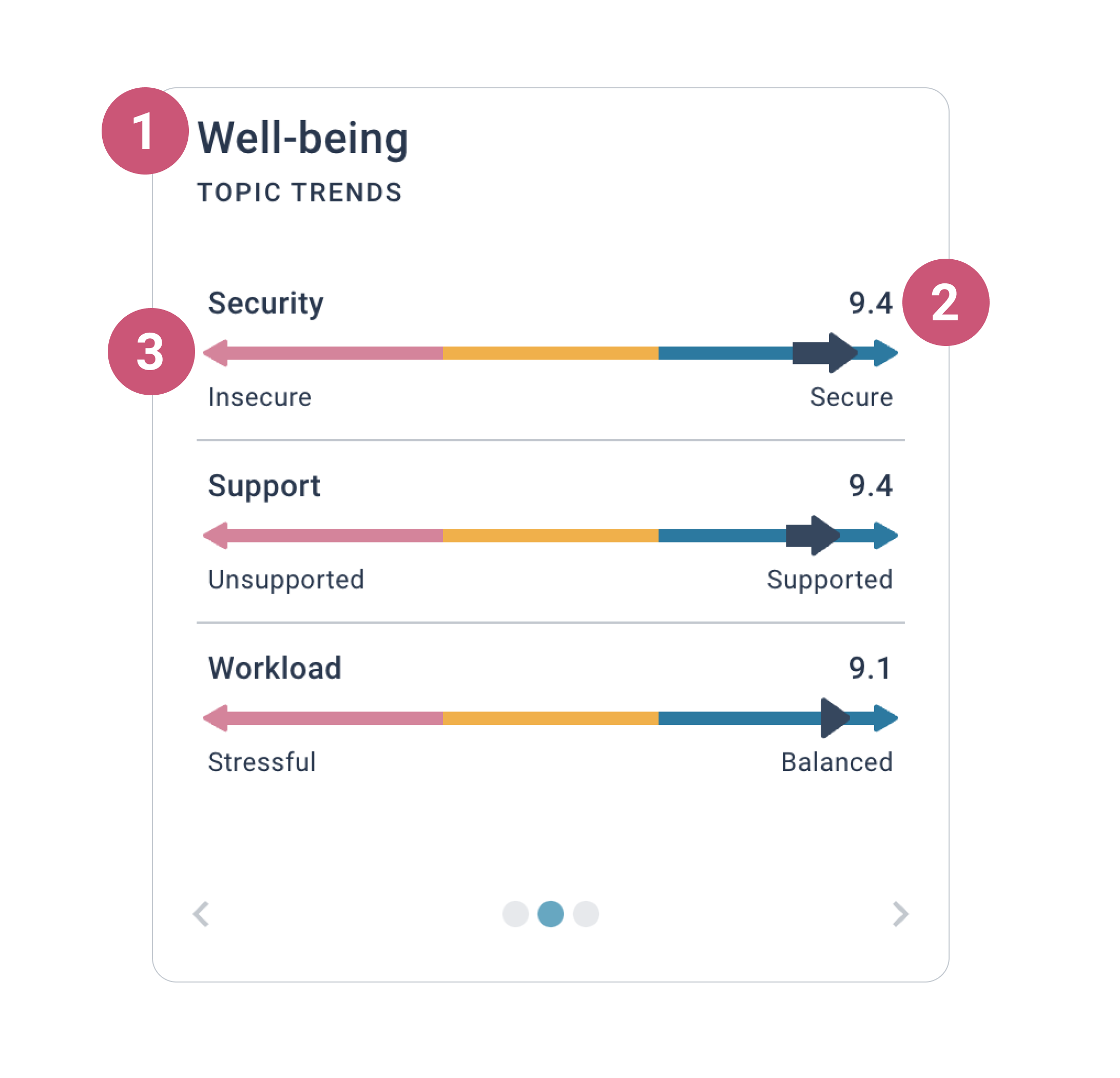1. Help Center
2. How to use Joyous
3. Understanding Insights

Understanding the topic trends

📺  Watch the video lesson here: Understanding insights

Topic trends let you see topics within a category and how those topics are trending. Trends provide clues to help you figure out which topics you might need to focus on.

What is shown in topic trends

Topic trends consist of three key pieces of information.1. The category name.

2. The average score for each topic.

• We use a simple average to calculate scores.
• Questions and topics are not weighted.
• Each individual rating contributes equally to an average score. For example:  (7 + 4 + 10 + 0 + 2 + 5) / 6 = 4.7

📝  Note: If the same question has been asked more than once over time, only the most recent one's ratings will be included in the average score calculation.

3. The trend for each topic.

• The trend line compares the average score of the last two questions asked for the topic.
• If the arrow is pointing to the right, there is a positive trend.
• If the arrow is pointing to the left, there is a negative trend.

💡 Tip: If a topic has a negative trend, you should view the low scoring questions relating to this topic on rated statements over time and jump to the related conversations for context on what you might want to focus on.

• If you see a dot, there is no change between the average score of the last two questions.